# CBSE 12th class Paper Solved – 2015-16 session

Sample Question Paper – Set II
Computer Science (083)
Class- XII (2015-16)
Time: 3hrs M.M: 70

Instructions:
i. All Questions are Compulsory.
ii. Programming Language: Section A : C++
iii. Programming Language: Section B: Python
iv. Answer either Section A or B, and Section C is compulsory

#### Section : A (C++)

Q1 a. Define Macro with suitable example. 2

Ans: Macros are preprocessor directive created using # define that serve as symbolic constants. They are a fragment of code which has been given a name. Whenever the name is used, it is replaced by the contents of the macro. They are created to simplify and reduce the amount of repetitive coding.
For instance,

 `#define sum (a, b) a + b`

Defines the macro sum, taking two arguments a and b. This macro may be called like any function. Therefore, after preprocessing:

 `z = sum(x, y); ` `is replaced with Z = x + y;`

b. Which C++ header file (s) will be included to run /execute the following C++ code? 1

 `void` `main() ` `{ ` `    ``int` `Last = 26.5698742658; ` `    ``cout << setw(5) << setprecision(9) << Last; ` `}`

Ans: The two header files that need to be included are:
iostream: iostream stands for standard input output stream. This header file contains definitions to objects like cin, cout etc.
iomanip: iomanip stands for input output manipulators. The methods declared in this files are used for manipulating streams. This file contains definitions of setw, setprecision etc.

c. Rewrite the following program after removing any syntactical errors. Underline each correction made. 2

 `#include ` `void` `main() ``int` `A; ` `A = [ 3, 2, 5, 4, 7, 9, 10 ]; ` `for` `(p = 0; p <= 6; p++) { ` `    ``if` `(A[p] % 2 = 0) ` `        ``int` `S = S + A[p]; ` `} ` `cout << S; ` `} `

Ans: In the above code, curly bracket after main() is missing, the elements of the array are wrongly declared, variable p is not declared and S can’t be declared inside the block. So, the following corrections are made:

```#include <iostream.h>
void main() {

int A = { 3, 2, 5, 4, 7, 9, 10 };
int S = 0, p;
for (p = 0; p <= 6; p++) {
if (A[p] % 2 == 0)
S = S + A[p];
}
cout << S;
}```

d. Find the output of the following C++ program: 2

 `#include ` `void` `repch(``char` `s[]) ` `{ ` `    ``for` `(``int` `i = 0; s[i] != ``'\0'``; i++) { ` `        ``if` `(((i % 2) != 0) && (s[i] != s[i + 1])) { ` `            ``s[i] = ``'@'``; ` `        ``} ` `        ``else` `if` `(s[i] == s[i + 1]) { ` `            ``s[i + 1] = ``'!'``; ` `            ``i++; ` `        ``} ` `    ``} ` `} ` `void` `main() ` `{ ` `    ``char` `str[] = ``"SUCCESS"``; ` `    ``cout << ``"Original String"` `<< str ` `    ``repch(str); ` `    ``cout << ``"Changed String"` `<< str; ` `} `

Ans: Output: Replace ‘@’ in place of U, ‘!’ in place of second C and ‘!’ in place of third S

```Original String SUCCESS
Changed String S@C!ES! ```

e. Find the output of the following : 3

 `#include ` ` `  `void` `switchover(``int` `A[], ``int` `N, ``int` `split) ` `{ ` ` `  `    ``for` `(``int` `K = 0; K < N; K++) ` `        ``if` `(K < split) ` `            ``A[K] += K; ` `        ``else` `            ``A[K] *= K; ` `} ` ` `  `void` `display(``int` `A[], ``int` `N) ` `{ ` `    ``for` `(``int` `K = 0; K < N; K++) ` `        ``(K % 2 == 0) ? cout << A[K] << ``"%"` `: cout << A[K] << endl; ` `} ` `void` `main() ` `{ ` `    ``int` `H[] = { 30, 40, 50, 20, 10, 5 }; ` `    ``switchover(H, 6, 3); ` `    ``display(H, 6); ` `} `

Ans: In the above program, when switchover () function is called by passing the address of array, size of array and split = 3. In this function for K = 0, 1, 2, if condition is executed and K is added to the array elements i.e. 30+0 = 30, 40+1 = 41, 50+2 = 52 and after that else condition is followed and array elements are multiplied, 20*3 = 60, 10*4 = 40, 5*5 = 25.
Display () prints the value of the modified array and the output is:

```30%41
52%60
40%25
```

f. Observe the following C++ code and find out, which out of the given options i) to iv) are the expected correct output. Also assign the maximum and minimum value that can be assigned to the variable ‘Go’. 2

 `void` `main() ` `{ ` `    ``int` `X = { 100, 75, 10, 125 }; ` `    ``int` `Go = random(2) + 2; ` `    ``for` `(``int` `i = Go; i < 4; i++) ` `        ``cout << X[i] << ``"\$\$"``; ` `}`

i. 100\$\$75 ii. 75\$\$10\$\$125\$\$ iii. 75\$\$10\$\$ iv.10\$\$125\$\$

Ans: Option (iv) can be the only correct answer if Go=2. In this case 10\$\$125\$\$ will be printed.
Minimum value of Go = 2 (when random(2) = 0)
Maximum value of Go = 3 (when random(2) = 1)

Q2 a. Differentiate between data abstraction and data hiding. 2
Ans: Data hiding can be defined as the mechanism of hiding the data of a class from the outside world. This is done to protect the data from any accidental or intentional access. Data hiding is achieved by making the members of the class private.
Data abstraction refers to, providing only essential information to the outside world and hiding their background details. Members defined with a public label are accessible to all parts of the program. The data abstraction view of a type is defined by its public members.

b. Answer the questions (i) and (ii) after going through the following class : 2

 `class` `Exam { ` `    ``int` `Rollno; ` `    ``char` `Cname; ` `    ``float` `Marks; ` ` `  `public``: ` `    ``Exam() ``// Function 1 ` `    ``{ ` `        ``Rollno = 0; ` `        ``Cname =``""``; ` `        ``Marks = 0.0; ` `    ``} ` `    ``Exam(``int` `Rno, ``char` `candname) ``// Function 2 ` `    ``{ ` `        ``Rollno = Rno; ` `        ``strcpy``(Cname, candname); ` `    ``} ` `    ``~Exam() ``// Function 3 ` `    ``{ ` `        ``cout << ``"Result will be intimated shortly"` `<< endl; ` `    ``} ` `    ``void` `Display() ``// Function 4 ` `    ``{ ` `        ``cout << ``"Roll no :"``<< Rollno; ` `        ``cout <<``"Name :"` `<< Cname; ` `        ``cout <<``" Marks :"``<< Marks; ` `    ``} ` `}; `

(i) Which OOP concept does Function 1 and Function 2 implement. Explain?
Ans: Function 1 and Function 2 are Constructor, which implement the concept Overloading and Polymorphism, as multiple definitions for Constructors are given in the same scope. Function 1 is a Default constructor and function 2 is a Parameterized constructor.

(ii) What is Function 3 called? When will it be invoked?
Ans: Function 3 is a Destructor which is invoked when the object goes out of scope.

c. Define a class Candidate in C++ with the following specification : 4
Private Members :

• A data members Rno(Registration Number) type long
• A data member Cname of type string
• A data members Agg_marks (Aggregate Marks) of type float
• A data members Grade of type char
• A member function setGrade () to find the grade as per the aggregate marks obtained by the student. Equivalent aggregate marks range and the respective grade as shown below:
>=80 A
Less than 80 and >=65 B
Less than 65 and >=50 C
Less than 50 D

Public members:

• A constructor to assign default values to data members: Rno=0, Cname="N.A", Agg_marks=0.0
• A function Getdata () to allow users to enter values for Rno. Cname, Agg_marks and call function setGrade () to find the grade.
• A function dispResult( ) to allow user to view the content of all the data members.

Ans: :

 `class` `Candidate { ` `    ``long` `Rno; ` `    ``char` `Cname; ` `    ``float` `Agg_marks; ` `    ``char` `Grade; ` `    ``void` `setGrade() ` `    ``{ ` `        ``if` `(Agg_marks >= 80) ` `            ``Grade = ‘A’; ` `        ``else` `if` `(Agg_marks < 80 && Agg_marks >= 65) ` `            ``Grade = ‘B’; ` `        ``else` `if` `(Agg_marks < 65 && Agg_marks >= 50) ` `            ``Grade =’C’; ` `        ``else` `            ``Grade =’D’; ` `    ``} ` ` `  `public``: ` `    ``Candidate() ` `    ``{ ` `        ``Rno = 0; ` `        ``Strcpy(Cname, ``"N.A."``); ` `        ``Agg_marks = 0.0; ` `    ``} ` `    ``void` `Getdata() ` `    ``{ ` `        ``cout << ``"Registration No"``; ` `        ``cin >> Rno; ` `        ``cout << ``"Name"``; ` `        ``cin >> Cname; ` `        ``cout << Aggregate Marks ";  ` `        ``cin >> Agg_marks; ` `        ``setGrade(); ` `    ``} ` `    ``void` `dispResult() ` `    ``{ ` `        ``cout << ``"Registration No"` `<< Rno; ` `        ``cout << ``"Name"` `<< Cname; ` `        ``cout << Aggregate Marks "<< Agg_marks;  ` `    ``} `

d. Give the following class definition answer the question that is follows: 4

 `class` `University { ` ` `  `    ``char` `name; ` ` `  `protected``: ` `    ``char` `vc; ` ` `  `public``: ` `    ``void` `estd(); ` `    ``void` `inputdata(); ` `    ``void` `outputdata(); ` `}  ` ` `  `class` `College : ``protected` `University {  ` ` `  `        ``int` `regno; ` `        ``protected` `        ``char` `principal() ` ` `  `public` `: ` `   ``int` `no_of_students; ` `   ``void` `readdata(); ` `   ``void` `dispdata ( ); ` `}; ` ` `  `class` `Department : ``public` `College { ` ` `  `             ``char` `name; ` `public``: ` `        ``void` `fetchdata(``int``); ` `        ``void` `displaydata();  }; `

i) Name the base class and derived class of college. 1
Ans:
Base class: University
Derived class: Department

ii) Name the data member(s) that can be accessed from function displaydata().
Ans: char name, char HOD, char principal(), int no_of_students, char vc

iii) What type of inheritance is depicted in the above class definition?
Ans: Multilevel Inheritance

iv) What will be the size of an object (in bytes) of class Department?
Ans: 85 bytes

Q3 a. An integer array A  is stored along the column in the memory. If the element A is stored at 50000, find out the location of A. 3
Ans:

A[i][j] = B + W[No.of rows x (I - Lr) + (J - Lc)]
A = B + 2[30 x (20 - 0) + (25 - 0)]
50000 = B + 2[30 x (20 - 0) + (25 - 0)]
B = 48750

A = 48750 + 2[30 x (7 - 0) + (10 - 0)]
A = 49190

b. Write the definition of functions for the linked implemented queue containing passenger information as follows: 4

 `struct` `NODE { ` `    ``int` `Ticketno; ` `    ``char` `PName; ` `    ``NODE* NEXT; ` `}; ` `class` `Queueofbus { ` `    ``NODE *Rear, *Front; ` ` `  `public``: ` `    ``Queueofbus() ` `    ``{ ` `        ``Rear = NULL; ` `        ``Front = NULL; ` `    ``}; ` `    ``void` `Insert(); ` `    ``void` `Delete(); ` `    ``~Queueofbus() ` `    ``{ ` `        ``cout << ``"Object destroyed"``; ` `    ``} ` `}; `

Ans:

 `void` `Queueofbus::Insert() ` `{ ` `    ``NODE* p = ``new` `NODE; ` `    ``cout << ``"Enter Ticket no"``; ` `    ``cin >> p->Ticketno; ` `    ``cout << ``"Enter Name"``; ` `    ``cin >> p->PName; ` `    ``p->NEXT = NULL; ` `    ``if` `(rear == NULL) { ` `        ``Rear = p; ` `        ``Front = Rear; ` `    ``} ` `    ``else` `{ ` `        ``Rear->NEXT = p; ` `        ``Rear = Rear->NEXT; ` `    ``} ` `} `

c. Write a function to sort any array of n elements using insertion sort. Array should be passed as argument to the function. 3

Ans:

 `void` `insertsort(``int` `a[], ``int` `n) ` `{ ` `    ``int` `p, ptr; ` `    ``// Assuming a = int_min i.e. smallest integer ` `    ``for` `(p = 1; p <= n; p++) { ` `        ``temp = a[p]; ` `        ``ptr = p - 1; ` `        ``while` `(temp <= a[ptr]) { ` `            ``a[ptr + 1] = a[ptr]; ``// Move Element Forward ` `            ``ptr--; ` `        ``} ` `        ``a[ptr + 1] = temp; ``// Insert Element in Proper Place ` `    ``}`

d. Write a function NewMAT(int A[][], int r, int c ) in C++, which accepts a 2d array of integer and its size as parameters divide all those array elements by 6 which are not in the range 60 to 600(both values inclusive) in the 2d Array . 2

Ans:

 `void` `NewMAT(``int` `A[][], ``int` `r, ``int` `c) ` `{ ` `    ``for` `(``int` `i = 0; i < r; i++) ` `        ``for` `(j = 0; j < c; j++) ` `            ``if` `((A[i][j] >= 60) && (A[i][j] <= 600)) ` `                ``A[i][j] /= 6; ` `    ``or A[i][j] = A[i][j] / 6; ` `} `

e. Evaluate the following postfix expression using stack and show the contents after execution of each Operations : 470, 5, 4, ^, 25, /, 6, *           2

Ans: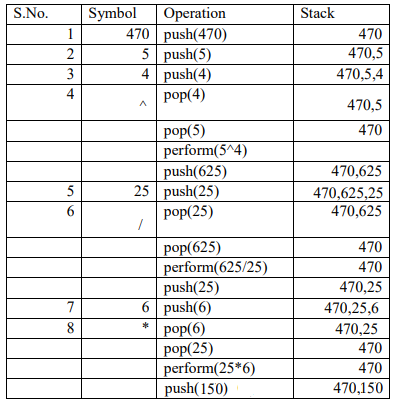Q4 a. Consider a file F containing objects E of class Emp. 1

i) Write statement to position the file pointer to the end of the file
Ans: F.seekg(0, ios::end);

ii) Write statement to return the number of bytes from the beginning of the file to the current position of the file pointer.
Ans: F.tellg();

b. Write a function RevText() to read a text file " Input.txt " and Print only word starting with ‘I’ in reverse order . 2
Example: If value in text file is: INDIA IS MY COUNTRY
Output will be: AIDNI SI MY COUNTRY

Ans:

 `void` `RevText() ` `{ ` `    ``ifstream in(``"Input.txt"``); ` `    ``char` `word; ` `    ``while` `(in) { ` `        ``in >> word; ` `        ``if` `(word ==’I’) ` `            ``cout << rrev(word); ` `        ``else` `            ``cout << word; `

c. Write a function in C++ to search and display details, whose destination is "Chandigarh" from binary file "Flight.Dat". Assuming the binary file is containing the objects of the following class: 3

 `class` `FLIGHT { ` ` `  `    ``int` `Fno;           ``// Flight Number ` `    ``char` `From;    ``// Flight Starting Point ` `    ``char` `To;     ``// Flight Destination ` `public``: ` `    ``char``* GetFrom(); ` `    ``{ ` `        ``return` `from; ` `    ``} ` `    ``char``* GetTo(); ` `    ``{ ` `        ``return` `To; ` `    ``} ` `    ``void` `input() ` `    ``{ ` `        ``cin >> Fno >> ; ` `        ``gets``(From); ` `        ``get(To); ` `    ``} ` `    ``void` `show() { cout Fno << ``": "` `From ``":"` `To endl; } ` `}; `

Ans:

 `void` `Dispdetails() ` `{ ` `    ``ifstream fin(``"Flight.Dat"``); ` `    ``Flight F; ` `    ``while` `(fin) { ` `        ``fin.read((``char``*)&F, ``sizeof``(F)) ``if` `(``strcmp``(F.GetTo(), ``"Chandigarh"``)) ` `            ``F.show(); ` `    ``} ` `} `

Section : B (Python)

Q1 a. List one similarity and one difference between List and Dictionary datatype. 2

Ans:

Similarity: Both List and Dictionary are mutable datatypes.
Dissimilarity: List is a sequential data type i.e. they are indexed.
Dictionary is a mapping datatype. It consists of key: value pair.
Eg: L =[1, 2, 3, 4, 5] is a list
D= {1:"Ajay", 2:"Prashant, 4:"Himani"} is a dictionary where 1, 2, 4 are keys and "Ajay", Prashant, "Himani" are their corresponding values.

b. Observe the following Python functions and write the name(s) of the module(s) to which they belong: 1
i) uniform() ii) findall()

Ans: i) random ii) re

c. Rewrite the following Python program after removing all the syntactical errors (if any), underlining each correction: 2

 `def` `checkval : x ``=` `raw_input``(``"Enter a number"``)  ` `if` `x ``%` `2` `=` `0` `:  ` `print` `x, ``"is even"`  `else` `if` `x < ``0` `:  ` `print` `x, ``"should be positive"`  `else``; ` `print` `x, ``"is odd"`

Ans:

```def checkval():
x = raw_input("Enter a number")
if x % 2 == 0:
print x, "is even"
elif x < 0 :
print x, "should be positive"
else:
print x, "is odd"
```

d. Find the output of the following Python program: 3

 `  `  `def` `makenew(mystr): ` `    ``newstr ``=` `" "` `    ``count ``=` `0` `    ``for` `i ``in` `mystr: ` `        ``if``(count ``%` `2` `!``=` `0``): ` `            ``newstr ``=` `newstr ``+` `str``(count) ` `        ``else``: ` `          ``if``(i.islower()): ` `              ``newstr ``=` `newstr ``+` `i.upper() ` `          ``else``: ` `              ``newstr ``=` `newstr ``+` `i  ` `        ``count ``+``=` `1` `    ``newstr ``=` `newstr ``+` `mystr[:``1``] ` `    ``print``(``"The new string is :"``, newstr) ` `makenew(``"sTUdeNT"``) `

Ans: The new string is: S1U3E5Ts

e. Find the output of the following program 2

 `def calcresult(): ` `    ``i = 9  ` `    ``while` `i > 1: ` `       ``if` `(i % 2 == 0): ` `          ``x = i % 2  ` `          ``i = i - 1  ` `       ``else``:  ` `          ``i = i - 2 ` `          ``x = i  ` `       ``print x * *2 `

Ans:

Output:

```49
25
9
1
```

f. Observe the following Python code and find out, which out of the given options i) to iv) are the expected correct output(s). Also assign the maximum and minimum value that can be assigned to the variable ‘Go’. 2

 `import` `random X ``=``[``100``, ``75``, ``10``, ``125``]  ` `Go ``=` `random.randint(``0``, ``3``) ` `for` `i ``in` `range``(Go) : ` `print` `X[i], ``"\$\$"``,  `

i. 100\$\$75\$\$10\$\$ ii. 75\$\$10\$\$125\$\$ iii. 75\$\$10\$\$ iv.10\$\$125\$\$100

Ans:
i. 100\$\$75\$\$10\$\$
Minimum Value that can be assigned to Go is 0
Maximum Value that can be assigned to Go is 3

Q2 a. Discuss the strategies employed by python for memory allocation? 2

Ans: Python uses two strategies for memory allocation- Reference counting and Automatic garbage collection:
Reference Counting: works by counting the number of times an object is referenced by other objects in the system. When an object's reference count reaches zero, Python collects it automatically.
Automatic Garbage Collection: Python schedules garbage collection based upon a threshold of object allocations and object de-allocations. When the number of allocations minus the number of deallocations are greater than the threshold number, the garbage collector is run and the unused block of memory is reclaimed.

b. Answer the questions (i) and (ii) after going through the following class definition: 2

```class Toy :
tid =0;
tcat = " "
def __init__(self):// Function1
10
..................................... // Blank 2```

i. Explain relevance of Function 1.

Ans: __init__ function is used to initialize the members of a class. It is automatically invoked when the object of the class is created.

ii (a). Fill in the blanks with a statement to create object of the class TOY.

Ans: T=Toy()

(b). Write statement to check whether tprice is an attribute of class TOY.

Ans: hasattr(T, tprice)

c. Define a class Train in PYTHON with following description: 4

Data Members
src of type string
Tnm of type string
dest of type string
charges of float
• A member function Getdata to assign the following values for Charges

Dest Charges
Mumbai 1000
Chennai 2000
Kolkata 2500

Public Members:
• A constructor to initialize the data members.
• A function InputData() to allow the user to enter the values
• A function displaydata() to display all and call getdata function

Ans:

 `class` `train :  ` `    ``def` `__init__(``self``): ` `        ``_src ``=` `""  ` `        ``_tnm ``=` `"" ` `        ``_dest ``=` `""  ` `        ``_charges ``=` `0.0` ` `  `    ``def` `getdata(``self``):  ` `        ``if` `self``._dest ``=``=` `"mumbai"` `or` `self``._dest ``=``=` `"MUMBAI"``: ` `           ``self``._charges ``=` `1000` `        ``elif` `self``._dest ``=``=` `"chennai"` `or` `self``._dest ``=``=` `"CHENNAI"` `:  ` `            ``self``._charges ``=` `2000` `        ``elif` `self``._dest ``=``=` `"kolkata"` `or` `self``._dest ``=``=` `"KOLKATA"` `:  ` `            ``self``._charges ``=` `2500` `     `  `    ``def` `inputdata(``self``):  ` `        ``self``._src ``=` `raw_input``(``"enter the source of journey"``)     ` `        ``self``._tnm ``=` `raw_input``(``"enter the train name"``) ` `        ``self``._dest ``=` `raw_input``(``"enter the destination"``)                                                  `

d. Observe the following class definition and answer the question that follow: 2

 `class ParentClass(objects): ` `    ``def__init__(self) ` `        ``self, x = 1 ` `        ``self.y = 10 ` `    ``def print(self): ` `        ``print(self.x, self.y) ` `class ChildClass(ParentClass): ` `    ``def__init__(self): ` `        ``super(ChildClass, self).init_() # Line 1 ` `        ``self, x = 2 ` `        ``self.y = 20 ` `c = ChildClass() ` `c.print()       `

i. Explain the relevance of Line1..

Ans: super() function is used to call the methods of base class which have been extended in derived class. Also, it is the importance of derived class __init__() to invoke the base class __init__().

ii. What type of inheritance is being demonstrated in the above code?

e. Write a user defined function findname(name) where name is an argument in Python to delete phone number from a dictionary phonebook on the basis of the name, where name is the key.

Ans:

 `def findname(name):  ` `     ``if phonebook.has_key(): ` `         ``del phonebook[name]  ` `     ``else:  ` `         ``print "Name not found"  ` `print "Phonebook Information" `

Q3 a. Explain try..except…else … with the help of user defined function def divide(x, y) which raises an error when the denominator is zero while dividing x by y and displays the quotient otherwise. 3

Ans:

 `def` `divide(x, y): ` `  ``try``: ` `    ``result ``=` `x ``/` `y ` `  ``except` `ZeroDivisionError: ` `    ``print` `"division by zero!"` `  ``else``: ` `    ``print` `"result is"``, result `

In the above example: try block consists of code that can raise an error. When y(denominator) gets a 0 value, ZeroDivisionError is raised which is handled by except clause. In case of no exception else statement is executed. In case there is no error the statement(s) in else clause are executed

b. Write a user defined function arrangelements(X), that accepts a list X of integers and sets all the negative elements to the left and all positive elements to the right of the list.
Eg: if L =[1, -2, 3, 4, -5, 7], the output should be: [-2, -5, 3, 4, 7]        3
Eg: if L =[1, -2, 3, 4, -5, 7], the output should be: [-2, -5, 3, 4, 7]        3

Ans:

 `def` `arrangelements(X): ` `    ``L ``=` `len``(X) ` `    ``for` `i ``in` `range``(L): ` `        ``if` `a[i] < ``0` `and` `i !``=` `0``: ` `            ``j ``=` `i ` `    ``while` `j !``=` `0` `and` `a[j ``-` `1``] > ``0``: ` `        ``a[j], [j ``-` `1``] ``=` `a[j ``-` `1``], a[j] ` `        ``j ``=` `j ``-` `1`

c. Consider the following class definition:-         3

 `class` `book (): ` `    ``bk ``=` `[] ` `    ``def` `_ init _ (``self``, bno): ` `        ``self` `.bno ``=` `bno ` `    ``def` `addbook (``self``): ` `        ``…………… ` `    ``def` `removebook (``self``): ` `        ``…………… `

The class book is implemented using Queue. Keeping the same in mind, complete the function definitions for adding a book addbook() and deleting a book removebook().

Ans:

 `def` `addbook(``self``): ` `    ``a ``=` `input``(``"enter book number: "``) ` `    ``book.bk.append(a) ` `    ``def` `removebook (``self``): ` `        ``if` `(book.bk ``=``=``[]): ` `            ``print` `"Queue empty"` `        ``else``: ` `            ``print` `"deleted element is: "``, book.bk[``0``] ` `del` `book.bk[``0``] `

d. Write a python function generate fibo(n) where n is the limit using a generator function Fibonacci (max) where max is the limit n that produces Fibonacci series. 3

Ans:

 `def` `Fibonacci (``max``): ` ` ``a, b ``=` `0``, ``1` `  ``while` `a <``=` `max``: ` `    ``yield` `a ` `      ``a, b ``=` `b, a ``+` `b ` ` ``def` `generatefibo(n) ` `   ``for` `i ``in` `Fibonacci (n): ` `     ``print` `i  `

e. Evaluate the following postfix using stack & show the content of the stack after the execution of each:        2

20, 4, +, 3, -, 7, 1

Ans: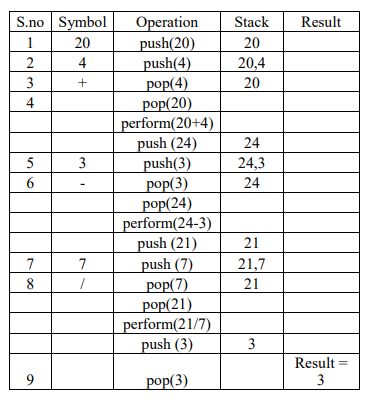Q4 a. Consider the following code: 1

 `f = open("mytry", "w+") ` `f.write("0123456789abcdef") ` `f.seek(-3, 2)   // 1 ` `printf.read(2) // 2 `

Explain statement 1 and give output of 2.

Ans: Statement 1 uses seek()method can be used to position the file object at a particular place in the file. It's syntax is :fileobject.seek(offset [, from_what]). So, f.seek(-3, 2) positions the fileobject to 3 bytes before the end of the file.

Output of 2 is: de (It reads 2 bytes from where the file object is placed.)

b. Write a user defined function in Python that displays the number of lines starting with ‘H’ in the file Para.txt.Eg: if the file contains: 2

Whose woods these are I think I know.
His house is in the village though;
He will not see me stopping here
To watch his woods fill up with snow.
Then the line count should be 2.

Ans:

 `def` `countH(): ` `  ``f ``=` `open` `(``"Para.txt"``, ``"r"``) ` `  ``lines ``=` `0` `  ``l ``=` `f.readlines() ` `  ``for` `i ``in` `l: ` `    ``if` `i[``0``] ``=``=` `'H'``: ` `       ``lines ``+``=` `1` `print` `"no. of lines is"``, lines `

c. Consider a binary file Employee.dat containing details such as empno: ename: salary (separator ‘ :’). Write a python function to display details of those employees who are earning between 20000 and 40000.(both values inclusive) 3

Ans:

 `def Readfile():  ` ` ``i = open("Employee.dat", "rb+") ` ` ``x = i.readline()  ` `    ``while (x): ` `        ``I = x.split(':')  ` `    ``if (20000 >= float(I) <= 5000):  ` `        ``print x ` `x = i.readline() `

Section : C

Q5 a. Differentiate between cardinality and degree of a table with the help of an example. 2

Ans:
Cardinality is defined as the number of rows in a table.
Degree is the number of columns in a table.

b) Consider the following tables FACULTY and COURSES. Write SQL commands for the statements (i) to (v) and give outputs for SQL queries (vi) to (vii) 6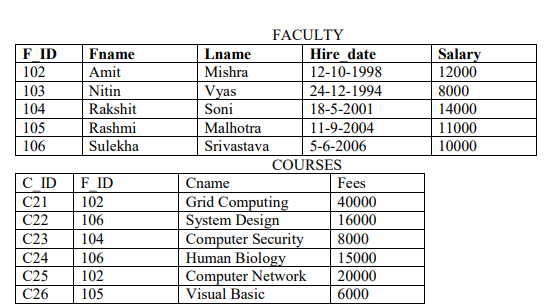i) To display details of those Faculties whose salary is greater than 12000.

Ans:

```Select * from faculty
where salary > 12000
```

ii) To display the details of courses whose fees is in the range of 15000 to 50000 (both values included).

Ans:

```Select * from Courses
where fees between 15000 and 50000
```

iii) To increase the fees of all courses by 500 of "System Design" Course.
Ans:

```Update courses set fees = fees + 500
where Cname = "System Design"
```

iv) To display details of those courses which are taught by ‘Sulekha’ in descending order ofcourses.

Ans:

```Select *from faculty fac, courses cour
where fac.f_id = cour.f_id and
fac.fname = 'Sulekha' order by cname desc
```

v) Select COUNT(DISTINCT F_ID) from COURSES;
Ans: 4

vi) Select MIN(Salary) from FACULTY, COURSES where COURSES.F_ID = FACULTY.F_ID;
Ans: 6000

Q6 a. State and Verify Absorption law algebraically. 2

Ans:

Absorption law states that:
A + AB = A and A.(A + B) = A

Algebraic method:
Taking LHS : A + AB
= (A.1) + (A.B) by Identity
= A. (1+B) by Distribution
= A.1 by Null Element
= A

b. Draw a logic circuit for the following Boolean expression: A.B+C.D’. 2

Ans: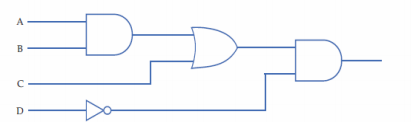c. Write the SOP form of a Boolean function F, which is represented in a truth table as follows: 1
A B C F
0 0 0 0
0 0 1 1
0 1 0 1
0 1 1 0
1 0 0 1
1 0 1 1
1 1 0 0
1 1 1 0

Ans: A'B'C + A'BC' + AB'C' + AB'C

d. Obtain a simplified from for a Boolean expression: F (U, V, W, Z) = II (0, 1, 3, 5, 6, 7, 15)

Ans: (u + v + w).(u + z').(v' + w').(u' + w' + z)

Q7 a. Write any 1 advantage and 1 disadvantage of Bus topology. 1

Ans:
Advantage: Since there is a single common data path connecting all the nodes, the bus topology uses a very short cable length which considerably reduces the installation cost.
Disadvantage: Fault detection and isolation is difficult. This is because control of the network is not centralized in any particular node. If a node is faulty on the bus, detection of fault may have to be performed at many points on the network. The faulty node has then to be rectified at that connection point.

b. SunRise Pvt. Ltd. is setting up the network in the Ahmadabad. There are four departments named as MrktDept, FunDept, LegalDept, SalesDept. 4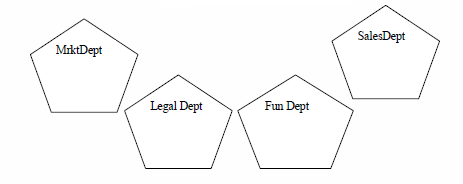Distance between various buildings is given as follows:

 MrktDept to FuncDept 80 m MrktDept to LegalDept 180 m MrktDept to SalesDept 100 m LegalDept to SalesDept 150 m LegalDept to FunDept 100 m FunDept to SalesDept 50 m

Number of Computers in the buildings:

 MrktDept 20 LegalDept 10 FunDept 08 SalesDept 42

i) Suggest a cable layout of connections between the Departments and specify topology.

Ans: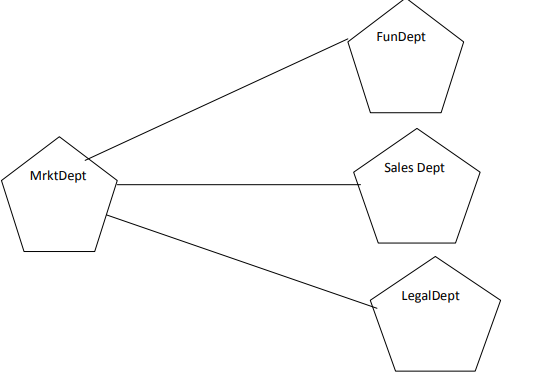ii) Suggest the most suitable building to place the server with a suitable reason.
Ans: As per 80 – 20 rule, MrktDept because it has maximum no. of computers.

iii) Suggest the placement of i) modem ii) Hub /Switch in the network.

Ans: Each building should have a hub/switch and Modem in case Internet connection is required

iv) The organization is planning to link its sale counter situated in various part of the same city/ which type of network out of LAN, WAN, MAN will be formed??Justify.

Ans: MAN (Metropolitan Area Network)

c. Name the protocol 1

i. Used to transfer voice using packet switched network.

Ans: VOIP (Voice Over Internet Protocol), is used for the transmission of voice traffic over Internet-based networks.

ii. Used for chatting between 2 groups or between 2 individuals.

Ans: Internet Relay Chat is an open protocol that allows users to chat with each other online. It operates on a client/server model.

d. What is an IP Address? 1
Ans: Internet Protocol (IP) Address is an address that uniquely identifies a device on the Internet. It allows a system to be recognized by other systems that are present on the world wide web. There are two primary types of IP address formats used — IPv4 and IPv6. Data transmission and routing over the internet requires the IP address of both the sender and receiver computer.

e. What is HTTP? 1

Ans: Hyper Text Transfer Protocol(HTTP) is an application-level protocol used to transfer data like text, graphic, images, sound, video, and other multimedia files over the web. HTTP is a part of the Internet protocol suite and uses a server-client paradigm for transmitting data.

f. Explain the importance of Cookies. 1

Ans: Cookies are small text files stored in the user's browser directory or data folder to retain the browsing information of a user like the login credentials, browsed web pages to identify customers and customize their browsing experience. The main purpose of a cookie is to identify users and possibly prepare customized Web pages or to save site login information for the user.

g. How is 4G different from 3G? 1

Ans: 3G stands for 3rd Generation and 4G is the 4th Generation of mobile broadband internet. The basic difference between the 3G and the 4G is the speed of transmission of mobile data. The average 3G speed is considered to be 3 MBps while in 4G, it is upto 15 MBps in India.

Attention reader! Don't stop learning now. Get hold of all the important DSA concepts with the DSA Self Paced Course at a student-friendly price and become industry ready.

My Personal Notes arrow_drop_upCheck out this Author's contributed articles.

If you like GeeksforGeeks and would like to contribute, you can also write an article using contribute.geeksforgeeks.org or mail your article to contribute@geeksforgeeks.org. See your article appearing on the GeeksforGeeks main page and help other Geeks.

Please Improve this article if you find anything incorrect by clicking on the "Improve Article" button below.

Article Tags :
Practice Tags :

Be the First to upvote.

Please write to us at contribute@geeksforgeeks.org to report any issue with the above content.